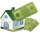# Expression of a variable from the formula + exponential equation - math problems

#### Number of problems found: 13

• Exponential equationFind x, if 625 ^ x = 5 The equation is exponential because the unknown is in the exponential power of 625
• DemographicsThe population grew in the city in 10 years from 30000 to 34000. What is the average annual percentage increase of population?
• What isWhat is the annual percentage increase in the city when the population has tripled in 20 years?
• Geometric progressionIn geometric progression, a1 = 7, q = 5. Find the condition for n to sum first n members is: sn≤217.
• DecibelBy what percentage does the sound intensity increase if the sound intensity level increases by 1 dB?
• Annual growthThe population has grown from 25,000 to 33,600 in 10 years. Calculate what was the average annual population growth in%?
• Growth of woodThe annual growth of wood in the forest is estimated at 2%. In how many years will make the forest volume double?
• Semiannually compound interestIf you deposit \$5000 into an account paying 8.25% annual interest compounded semiannually, how long until there is \$9350 in the account?
• Exponential warmSuppose that a body with temperature T1 is placed in surroundings with temperature T0 different from that of T1. The body will either cool or warm to temperature T(t) after time t, in minutes, where T(t)=T0 + (T1-T0)e^(-kt) . If jello salad with 30 degree
• Mortage hypo loanThe Jonáš family decided to buy an older apartment, which cost EUR 30,000. They found EUR 17,000 and took the loan with the bank for the remaining amount. What interest did they receive if they repay this amount for 15 years at EUR 120 per month?
• Before yesterdayHe merchant adds a sale sign in his shop window to the showed pair of shoes in the morning: "Today by p% cheaper than yesterday. " After a while, however, he decided that the sign saying: "Today 62.5% cheaper than the day before yesterday". Determine the
• Volume of woodEvery year, at the same time, an increase in the volume of wood in the forest is measured. The increase is regularly p% compared to the previous year. If in 10 years the volume of wood has increased by 10%, what is the number p?
• SavingsThe depositor regularly wants to invest the same amount of money in the financial institution at the beginning of the year and wants to save 10,000 euros at the end of the tenth year. What amount should he deposit if the annual interest rate for the annua

We apologize, but in this category are not a lot of examples.
Do you have an interesting mathematical word problem that you can't solve it? Submit a math problem, and we can try to solve it.

We will send a solution to your e-mail address. Solved examples are also published here. Please enter the e-mail correctly and check whether you don't have a full mailbox.

Please do not submit problems from current active competitions such as Mathematical Olympiad, correspondence seminars etc...

Expression of a variable from the formula - math word problems. Exponential equation - math word problems.